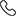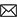Sales :9214233303 | 7240013135 / Tech. Support Desk :7231890000 (9AM ~ 6PM)# Differential Equations: All about its order, degree, application and more

29 Nov, 2022, By Tanvi SharmaAn equation that contains one or more functions with their derivatives is known as a differential equations. The derivatives indicate the rate at which the functions change at a point.  It is predominantly used in fields like Physics, Engineering, and Biology. The key purpose of the differential equation is the examination of the solution that sits well with the equation and properties of the solution.

The widely feared differential equation is not as difficult. With the usage of explicit formulas given in this article, you can easily solve this type of equation. Let’s discuss the definition, types, and methods to solve the differential equation, the order, and degree of the differential equation, ordinary differential equations with real-world examples, and a solved problem.

Also check: Complete mathematics course

## What is a differential equation?

An equation that contains one or more terms and the derivatives of a single variable in regard to the other variable.

dy/dx = f(x)

In the given equation ‘X’ is an independent variable and ‘Y’ is a dependent variable.

For example, dy/dx = 5x

A differential equation comprises derivatives that are either partial or ordinary derivatives. The derivatives represent a change in the rate, while a differential equation illustrates the relationship between the quantity that is constantly changing in regard to the change in the other quantity. You can use a variety of differential equation formulas to find solutions to the derivatives.

Also read: How to prepare for JEE without coaching

## Differential equation: The order

The highest order of the derivative present in the equation is the order of a differential equation. Given are some of the examples of different orders of the differential equations:

• dy/dx = 3x + 2 , the equation is in the order 1

• (d2y/dx2)+ 2 (dy/dx)+y = 0. The order is 2

• (dy/dt)+y = kt. The order is 1

### First order of the differential equation

As shown in example 1, the first order of the differential equation has a degree equal to 1. The linear equations included in the form of derivatives in the equation are in the first order. It comprises a single derivative such as dy/dx, where X and Y are the two variables, which is shown as:

dy/dx = f(x, y) = y’

### Second order of the differential equation

The equation that comprises a second-order derivative is referred to as a second-order differential equation.

The second-order differential equation is written as:

d/dx(dy/dx) = d2y/dx2 = f”(x) = y”

Also Check: The best timetable for JEE aspirants

### The Degree of differential equation

The power of the highest-order derivative wherein the original equation is characterized in the form of a Polynomial equation in the derivatives like y’,y”, y”’, and so on, is directed as the degree of the differential equation.

Example:

Assume (d2y/dx2)+ 2 (dy/dx)+y = 0 to be a differential equation, therefore the degree of the equation presented here is 1.

Given are some more examples of the same:

• dy/dx + 1 = 0, degree is 1

• (y”’)3 + 3y” + 6y’ – 12 = 0, the degree is 3.

• (dy/dx) + cos(dy/dx) = 0; the given is not a polynomial equation in y’ and the degree of such a differential equation cannot be explained.

Note: The order and degree are always positive integers of a differential equation.

Also check: Learning resources for JEE

### How many types of differential equations are there?

Differential equation can be classified into the following categories:

• Ordinary Differential Equation

• Partial Differential Equation

• Linear Differential Equation

• Nonlinear differential Equation

• Homogeneous Differential Equation

• Nonhomogeneous Differential Equation

Also read: Importance of mathematics in JEE Mains exam

### Differential Equation Solutions

The solution of the differential equation is the function that satisfies the given differential equation. Whereas, a solution that contains several arbitrary constants as the order of a differential equation is referred to as a general solution.

A solution that is unchained from arbitrary constants is called a particular solution.

There are two ways through which you can find a solution to a differential solution:

1. Separation of variables

2. Integrating factor

Also check: Important chapters for JEE exam by NTA

#### Separation of variables

This approach is used when the differential equation can be written as dy/dx = f(y)g(x) where the function of y is f and the function of x is g. Considering this as an initial condition, note this problem again as 1/f(y)dy= g(x)dx and then integrate on both sides.

#### Integrating factor

This technique is put into use when the differential equation is in the form of dy/dx + p(x)y = q(x) where p and q both are the functions of x only.

The first order of the differential equation is shown as y’+ P(x)y = Q(x). where x has p and q as its functions and the first derivative of y.

An equation that contains derivatives of an unknown factor that can be either a partial or ordinary derivative is known as a high-order differential derivative. It can be presented in any order.

Also check: JEE Main 2023 registration process

#### Applications of Differential equation

Differential equations have their uses in several fields such as applied mathematics, science, and engineering. Apart from its uses in the technical field, it is also used to deal with real-world problems.Let's familiarize ourselves with the uses of differential equations in real life:

1. It is used in describing various exponential growth and decay.

2. The differential equation is helpful in calculating the return on investment over a time period.

3. It is of major use in medical science in estimating the growth of cancer and the rolling of disease in the body.

4. It is also used in describing the movement of electricity.

5. Economists use it in discovering the optimum investment strategist.

6. Differential equations are helpful in describing the motion of waves or pendulums.

Also check: Difference between JEE and IIT

The other areas of its usage are heat conduction analysis, and understanding the motion of waves. The ordinary differential equation can be used in the field of engineering for finding the relationship between various parts of the bridge.

Keep following EtoosIndia JEE Articles for the latest updates.